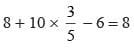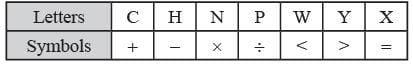# Olympiad Test: Mathematical Operations - Class 9

Test Description

## 15 Questions MCQ Test Science Olympiad Class 9 - Olympiad Test: Mathematical Operations

Olympiad Test: Mathematical Operations for Class 9 2023 is part of Science Olympiad Class 9 preparation. The Olympiad Test: Mathematical Operations questions and answers have been prepared according to the Class 9 exam syllabus.The Olympiad Test: Mathematical Operations MCQs are made for Class 9 2023 Exam. Find important definitions, questions, notes, meanings, examples, exercises, MCQs and online tests for Olympiad Test: Mathematical Operations below.
 1 Crore+ students have signed up on EduRev. Have you?
Olympiad Test: Mathematical Operations - Question 1

### If A denotes multiplication, B denotes addition, C denotes division and D denotes subtraction, then what is the value of 14 A 6 B 8 C 2 D 12?

Detailed Solution for Olympiad Test: Mathematical Operations - Question 1

Here, 14 A 6 B 8 C 2 D 12
= 14 × 6 + 8 ÷ 2 – 12
= 14 × 6 + 4 – 12
= 84 + 4 – 12
= 88 – 12 = 76

Olympiad Test: Mathematical Operations - Question 2

### If × denotes –; ÷ denotes +, + denotes ÷ and – denotes ×, which one of the following equation is correct?

Detailed Solution for Olympiad Test: Mathematical Operations - Question 2

(a) 15 – 5 ÷ 5 × 20 + 10 = 6
15 × 5  + 5 – 20 ÷ 10 = 6
15 × 5  + 5 – 2 = 6
78 ≠ 6
(b) 8 ÷ 10 – 3 + 5 × 6 = 8
8 + 10 × 3 ÷ 5 – 6 = 88 + 6 – 6 = 8
8 =  8
Hence (b) is true.

Olympiad Test: Mathematical Operations - Question 3

### If P means ×, R means +, T means ÷, S means –, then what is the value of 8 P 4 R 28 T 7 S 15?

Detailed Solution for Olympiad Test: Mathematical Operations - Question 3

Given 8 P 4 R 28 T 7 S 15
= 8 × 4 + 28 ÷ 7 – 15 = 8 × 4 + 4 – 15
= 32 + 4 – 15 = 36 – 15 = 21

Olympiad Test: Mathematical Operations - Question 4

If numbers 3 and 6 are interchanged and signs – and × are interchanged then which of the following is correct?

Detailed Solution for Olympiad Test: Mathematical Operations - Question 4

Given 3 × 6 – 4 = 6 – 3 × 4
= 6 – 12 = – 6 ≠ 33
6 × 3  – 4 = 3 – 6 × 4 = 3 – 24 = – 21 ≠ 15
3 – 6 × 8 = 6 × 3 – 8 = 18 – 8 = 10 = 10
∴ (c) is correct.

Olympiad Test: Mathematical Operations - Question 5

If A stands for +, B stands for –, C stands for ×. Then what is the value of (10 C 4) A (4 C 4) B 6?

Detailed Solution for Olympiad Test: Mathematical Operations - Question 5

We have (10 C 4) A (4 C 4) B 6
= (10 × 4) + (4 × 4) – 6
= 40 + 16 – 6 = 56 – 6 = 50

Olympiad Test: Mathematical Operations - Question 6

If A + B = 2C & C + D = 2A then which of the following is correct?

Detailed Solution for Olympiad Test: Mathematical Operations - Question 6

Here A + B = 2C …(1)
C +  D = 2A …(2)
A + B  + C + D = 2C + 2A
B + D = C + A

Olympiad Test: Mathematical Operations - Question 7

If L stands for +, M stands for –, N stands for × and P stands for ÷ then what is the value of 37 N 10 L 65 P 13 M 16?

Detailed Solution for Olympiad Test: Mathematical Operations - Question 7

Here 37 N 10 L 65 P 13 M 16
= 37 × 10 + 65 ÷ 13 – 16
= 37 × 10 + 5 – 16 = 370 + 5 – 16
= 370 – 11 = 359

Olympiad Test: Mathematical Operations - Question 8

If P stands for addition, L for division, M for subtraction and Z for multiplication then what is the value of 182 P 117 L 13 M 7 Z 16?

Detailed Solution for Olympiad Test: Mathematical Operations - Question 8

Here 182 P 117 L 13 M 7 Z 16
= 182 + 117 ÷ 13 – 7 × 16
= 182 + 9 – 7 × 16
= 182 + 9 – 112 = 70 + 9 = 79

Olympiad Test: Mathematical Operations - Question 9

If × stands for addition, ‘–’ for division, ÷ for subtraction & ‘+’ for multiplication. Then which of the following statement is correct?

Detailed Solution for Olympiad Test: Mathematical Operations - Question 9

4 × 5 + 9 – 3 ÷ 4 = 15
= 4 + 5 × 9 ÷ 3 – 4
= 4 + 5 × 3 – 4
= 4 + 15 – 4
= 15 = 15
∴ (d) is correct.

Olympiad Test: Mathematical Operations - Question 10

If ‘<’ stands for minus, ‘–’ stands for equal to ‘>’ stands for multiplication, ‘÷’ stands for greater than, ‘×’ for addition, ‘+’ stands for division, ‘=’ stands for less than then which of the following statement is correct?

Detailed Solution for Olympiad Test: Mathematical Operations - Question 10

Let 5 > 2 × 1 – 3 > 4 < 1
⇒ 5 × 2 + 1 = 3 × 4 – 1
⇒ 11 = 12 – 1
⇒ 11 =  11

Olympiad Test: Mathematical Operations - Question 11

Direction: If > denotes +, + denotes ÷, – denotes =, × denotes > and = denotes <, < denotes – and ↑ denotes × then choose the correct statement in each of the following problems :

Detailed Solution for Olympiad Test: Mathematical Operations - Question 11

Let 35 > 3 < 2 = 4 > 8 ↑ 7
⇒ 35 + 3 – 2 < 4 + 8 × 7
⇒  35 + 1 < 4 + 56
⇒ 36 < 60
∴ (a) is correct.

Olympiad Test: Mathematical Operations - Question 12

Direction: If > denotes +, + denotes ÷, – denotes =, × denotes > and = denotes <, < denotes – and ↑ denotes × then choose the correct statement in each of the following problems :

Detailed Solution for Olympiad Test: Mathematical Operations - Question 12

Let 4 > 6 + 2 × 32 + 4 < 1
4 + 6  ÷ 2 > 32 ÷ 4 – 1
4 + 3  > 8 – 1
7 = 7

Olympiad Test: Mathematical Operations - Question 13

Direction: In these question the different alphabets stand for various arithmetical and logical symbols. We have to choose the correct alternative according to given letter symbols.Detailed Solution for Olympiad Test: Mathematical Operations - Question 13

Let 5 Y 4 N 3 C 5 N 3 H 11
⇒ 5 < 4 × 3 + 5 × 3 – 11
⇒ 5 < 12 + 15 – 11
⇒ 5 < 16

Olympiad Test: Mathematical Operations - Question 14

Direction: In these question the different alphabets stand for various arithmetical and logical symbols. We have to choose the correct alternative according to given letter symbols.Detailed Solution for Olympiad Test: Mathematical Operations - Question 14

Here 6 P 3 C 4 N 3 Y 7 N 3
⇒ 6 ÷ 3 + 4 × 3 < 7 × 3
⇒ 2 + 4 × 3 < 21
⇒ 2 + 12 < 21
⇒ 14 <  21

Olympiad Test: Mathematical Operations - Question 15

Direction: In these question the different alphabets stand for various arithmetical and logical symbols. We have to choose the correct alternative according to given letter symbols.Detailed Solution for Olympiad Test: Mathematical Operations - Question 15

Here
14 C 13 P 13 N 6 H 5 W 2 C 12 P 3 N 2
⇒ 14 + 13 ÷ 13 × 6 – 5 > 2 + 12 ÷ 3 × 2
⇒ 14 + 1 × 6 – 5 > 2 + 4 × 2
⇒ 20 – 5 > 2 + 8
⇒ 15 > 10